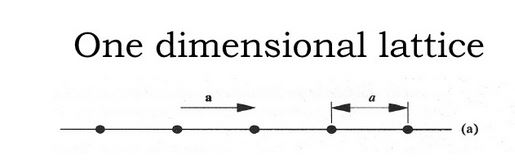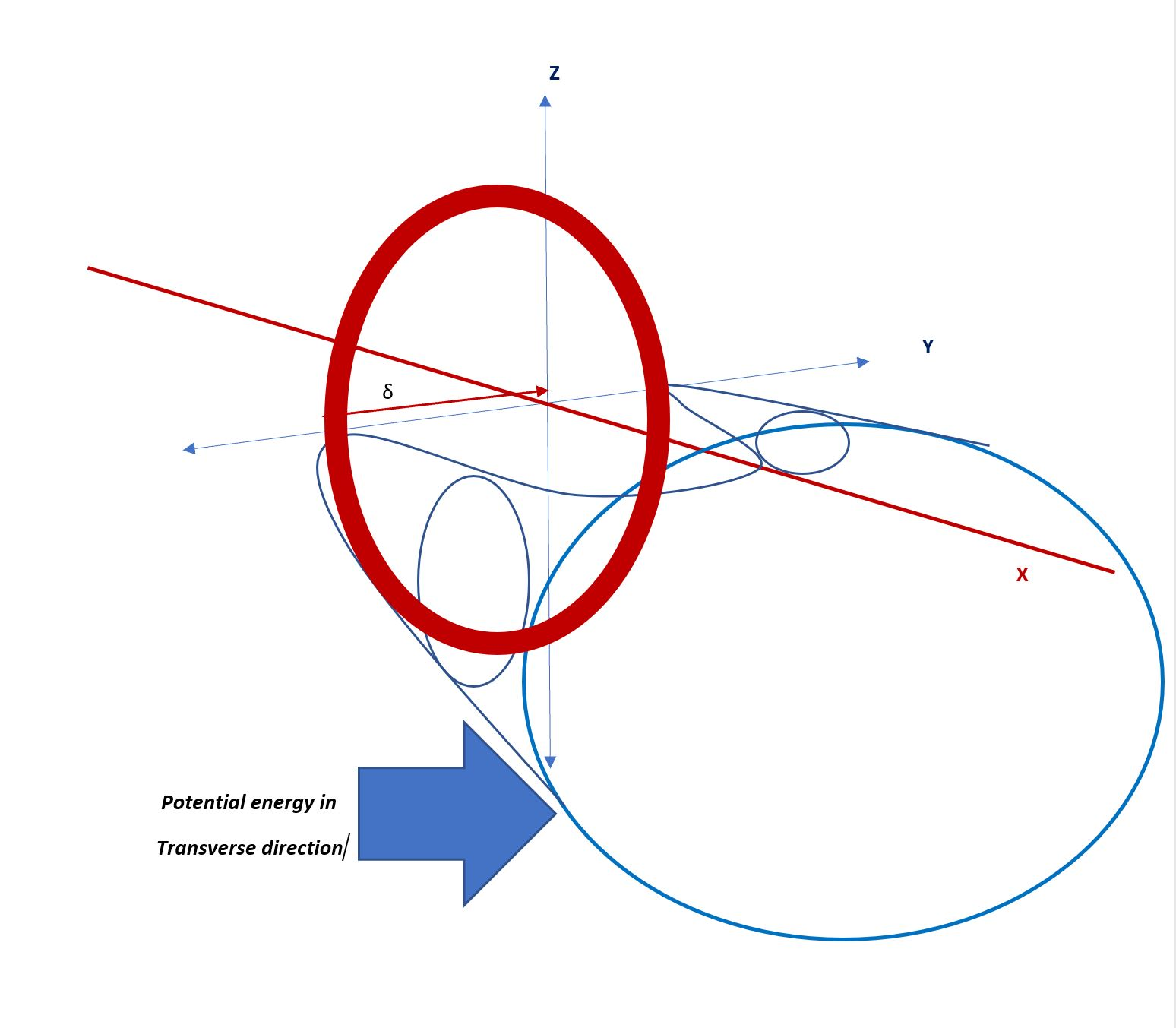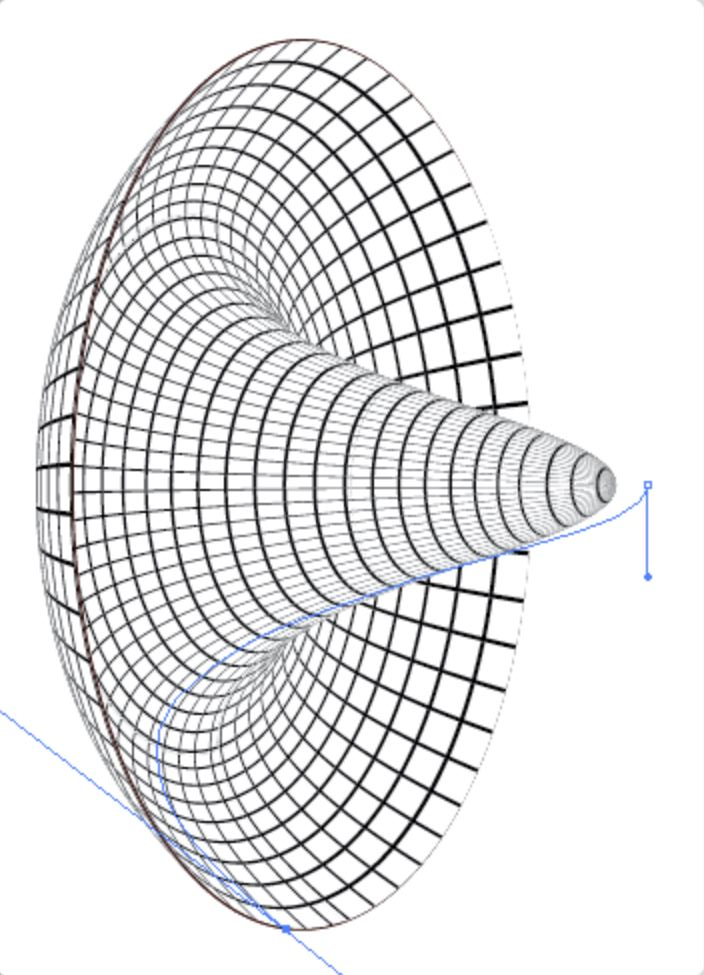# Gauge invariance, Global and Local Symmetry

This post, aimed at people with some knowledge of Maxwell’s equations, is aimed at connecting a bunch of concepts that are all central to how we understand the universe today. Nearly every word in the title has the status of being a buzz-word, but for good reason – they help organize the ideas well. Some of these ideas are being challenged, but nothing concrete has emerged as a convincing alternative.

When you study Maxwell’s equations in a sophomore course in college, you are presented with something like$\vec \nabla . E = \frac{\rho}{\epsilon_0} \: \: , \: \: \vec \nabla \times \vec E = - \frac{\partial \vec B}{\partial t} \: \: , \: \: \vec \nabla . \vec B = 0\: \: , \: \: c^2 \vec \nabla \times \vec B = \frac{\vec j}{\epsilon_0} + \frac{\partial \vec E}{\partial t}$

You are then told that these equations can be much simplified by introducing something called vector and scalar potentials ($\vec A, \phi$), such that$\vec B = \vec \nabla \times \vec A$ and$\vec E = - \vec \nabla \phi - \frac{1}{c} \frac{\partial \vec A}{\partial t}$.

Then we discover this peculiar property (I say this the way I first heard of it): you can amend these potentials in the following way$\vec A \rightarrow \vec A - \vec \nabla \chi \: \: , \: \: \phi \rightarrow \phi + \frac{1}{c} \frac{\partial \chi}{\partial t}$ for${\bf any}$ field$\chi$ and the physically measurable electric ($\vec E$) and magnetic fields ($\vec B$ ) are${\bf completely}$ unchanged., so are Maxwell’s equations.

I presume when Maxwell discovered this property (invariance of the electric and magnetic fields upon a “gauge transformation”), which was later named “gauge invariance”, it seemed like a curiosity. The vector potential seems to have a redundancy – it is only relevant up to the addition of$\vec \nabla \chi$ for any$\chi$ (and in conjunction with the scalar potential). But the physical properties of matter and charge only care about the electric and magnetic fields, which are insensitive to the above freedom. If you solve for the vector and scalar potential that applies to a particular problem, you can compute the electric/magnetic fields and go on without thinking about the potentials again.

Quantum mechanics was the first blip on the sedate view above. It turns out that a charged particle notices the vector potential, in a typically beautiful way (in Nature) that both preserves the above (gauge) redundancy, but is still noticeable in its physical effects. We could have a magnetic field far far away, but the vector potential that produces that magnetic field could exist here, close to an electron and there would be measurable effects due to that far away magnetic field. It is called the Aharonov-Bohm effect, but it is a standard feature of quantum mechanics. The vector potential seems to have physical significance, but there is the curious question of what gauge invariance actually means. And the correct quantum mechanical version of Schrodinger’s equation in the presence of an electromagnetic field is not$\frac{1}{2m} (-i \hbar \frac{\partial }{\partial x})(-i \hbar \frac{\partial }{\partial x}) \psi + V(x) \psi = E \psi$,

but$\frac{1}{2m} (-i \hbar \frac{\partial }{\partial x} - q A_x)(-i \hbar \frac{\partial }{\partial x} - q A_x) \psi + V(x) \psi = E \psi$,

This is all we have to go on.

If we require this “gauge-invariance” to be a generic property of electromagnetism, it implies that the energy must be a simple function of the physically relevant$\vec E$‘s and$\vec B$‘s, not some term involving$\vec A$‘s that might not be gauge-invariant. No term like$\vec A. \vec A$ can appear in the energy, because such a term would not be the same after you perform a gauge transformation on$\vec A$, i.e., add a$\vec \nabla \chi$ term as in the above. So what? This will become clear in the next paragraphs.

Let’s leave this aside for a bit.

The next big development in the theory of fundamental physics was quantum field theory. This theory has many things going for it, but the initial motivation was the discovery that particles can be created and destroyed, seemingly out of pure energy (or photons) and what’s more, every particle of a particular type is precisely identical to any other particle of the same type. All electrons look/feel the same and behave in exactly the same way. Whilst we have no idea why this is the way things are, we can model this behavior very nicely. The theory of quantum mechanics started out by considering a simple harmonic oscillator. The energy of the harmonic oscillator is$E = \frac{p^2}{2m} + \frac{m \omega^2 x^2}{2}$, where$\omega=$ the frequency of the oscillator,$m=$ the mass of the thing that’s oscillating while$x, p$ are the position and momentum of the thing that is oscillating.

It turns out that one can think of states of higher and higher energy of the harmonic oscillator as having more and more “quanta” of energy, since energy seems to be absorbed in packets (this is what was discovered in the quantum theory). These quanta appear identical to each other. If you have these harmonic oscillators at every point in space, then we have a “field” of oscillators. Then, with some simple construction, the “quanta” that are constructed from these several harmonic oscillators can be given a charge and a mass. The energy of this field can be written, for a field in only one dimension (the$x$-dimension) as$E = \frac{1}{2}(\frac{\partial \phi}{\partial x})^2 + \frac{1}{2} m^2 \phi^2$ :  there is a “strain energy” that’s the first term and a “mass” energy, which is related to the second term. We can treat the universe as being composed of these “fields”, though the universe seems to have scalar, vector, spinor and tensor fields too. They can each be mapped onto all known particles, so we can invent a quantum field for every single type of fundamental particle. Voila, all these particles are identical to each other and have exactly the same properties  – they are just “quanta” of these fields. Studying the properties of these particles is, basically, the business of quantum field theory.

If we had a field that wasn’t a scalar$\phi$, but a vector$\vec A$, the corresponding mass term would be a coefficient times$\vec A. \vec A$. But we already rejected such a term as it wouldn’t preserve gauge invariance, described in the above. You might then persist, to ask why we should try to preserve gauge invariance. Let me come back to this point a little further on. Right now, let me just say that the Aharanov-Bohm effect already tells us that Nature appears to care about this fig-leaf. In particular, it seemed for a long time that it was not possible to write a gauge-invariant theory of a massive (i.e,. not massless) particle described by a vector field.

The simplest kind of field is a scalar field, something akin to the temperature inside every point in a room. There is one number attached to every point in space.

Let’s simplify this to a simple collection of balls and springs. We could do this in any number of dimensions, but to start, let’s do this in one-dimension.Imagine little springs connecting the balls on the lattice. At equilibrium, the balls like to be equidistant from each other, separated by the lattice constant “a”. The “field”$\phi$ at every point is the displacement of the ball from the equilibrium point. The equilibrium value of this “field” is$0$ at every point.

We can express this by saying that each ball is sitting in a potential energy well along the transverse directions (perpendicular to the one-dimensional lattice) that looks like thiswhich has the result that the ball doesn’t like to roll away from the lattice point it is supposed to be in.

Now, suppose there is an additional wrinkle in this lattice. Let’s assume each ball has a small charge. That doesn’t change the equilibrium situation, since like charges repel and the balls would still keep a distance “a” apart (don’t forget there are still the springs holding the balls together) .

Suppose, now, that there is a small fuzzy charge donut around each ball, with radius$\delta$, and assume the charge in the fuzzy donut is small and opposite to that of the ball. In that case, the ball is attracted away from its equilibrium position and would rather sit at the point$\delta$ away from its usual position on the lattice.

The potential energy of each ball went from being zero, centered at the lattice point, to being positive (at the lattice site) with minima a little radius$\delta$ around the lattice point. This may be called a “broken” symmetry phase, the symmetry of the smooth parabola is replaced with this “Mexican hat”.

The position of the ball can be specified by a complex number now, it is$|\phi|$, the along-the-chain displacement of the ball times$e^{i \theta}$, where$\theta$ is the angular position on the donut shaped equilibrium surface. Why complex? Its just a simple representation of the position of the ball with this particular geometry.Another depiction of the potential is hereand the chain isIts not the particular mathematical function that is relevant here, just the idea that this sort of shape-shifting can happen due to natural evolution of parameters in the potential energy function, as we change external conditions.

But let’s assume the springs are pretty taut and hard to pull apart – when one ball decides to move to this new minimum, the others will all follow. This is a global shift of the entire system to the new potential energy well.  But where in this new well? There is a minimum all the way around and chain of balls could line up, ramrod straight, with all the balls on the same corresponding spot on the Mexican hat potential, at any point on the channel around the central hat.

The energy function of the scalar field in this case is$E = \frac{1}{2} \frac{\partial \phi^{*}}{\partial x} \frac{\partial \phi}{\partial x} {\bf -} \frac{1}{2} \mu^2 \phi^{*} \phi + \frac{\lambda}{4} (\phi^{*} \phi)^2$ or some similar function. Remember that$\phi$ is complex and we have just written this in a form that gives a “real” number for the energy. In the particular case of this function, the new equilibrium would be$|\phi|=\sqrt{\frac{\mu^2}{\lambda}}$; remember$|\phi|$ represents how far the ball is from the lattice point it started out at, so to choose a concrete spot,$x=0, y= \sqrt{\frac{\mu^2}{\lambda}}, z=0$. And the “global” symmetry here is that we could change the angular position$\theta$ of${\bf all}$ the balls and it wouldn’t affect the total energy of the system.

Now, for a more daring and remarkable idea, which undoubtedly only came about because Chen Ning Yang and Robert Mills were playing around with the idea of gauge invariance before they came to this realization.

Suppose we let each ball be in any spot it wants to be in that channel (donut shaped minimum) of the Mexican hat potential, but we require that the energy of the total system be the same. In that case, remember there are springs between the balls! They don’t want the balls to get far apart – they need to be allowed to relax a bit.

Concretely, we are saying, let$\theta$ be a function of$x$. If so, we have a problem. The terms proportional to$\phi^{*} \phi$ are unaffected, since they remain$|\phi|^2 e^{i \theta} e^{-i \theta} = |\phi|^2$. So are the terms proportional to$(\phi^{*} \phi)^2$.  Not so for the “strain” energy terms – the springs doth protest!$\frac{1}{2} \frac{\partial \phi^{*}}{\partial x} \frac{\partial \phi}{\partial x}$

which was$\frac{1}{2} \frac{\partial |\phi|}{\partial x} \frac{\partial |\phi|}{\partial x}$ when$\theta$ are independent of$x$ becomes, instead,$\frac{1}{2} (\frac{\partial |\phi|}{\partial x}- i |\phi| \frac{\partial \theta}{\partial x}) (\frac{\partial |\phi|}{\partial x}+ i |\phi| \frac{\partial \theta}{\partial x})$

This seems like a disaster for this idea – except that the idea that rescues it is to say, there is a field (let’s for reason of lack of imagination, call it$A$) that has does two things

• enters into the energy expression through the derivative term, i.e.,$\frac{\partial \phi}{\partial x} - i q A \phi$.
• has a property that the field$A$ has a peculiar kind of freedom – we can change$A$ to$A + \frac{\partial \chi}{\partial x}$.

But this is exactly the way the vector potential appears in the energy function and Schrodinger’s equation of a charged particle in an electromagnetic field, as was noted a few paragraphs above! With such a field, we can make a “gauge transformation” by$\frac{\partial \theta}{\partial x}$ and “remove” the effect of the spatially varying$\theta$ term. The “gauge field”$A$ allows you to turn the global symmetry into a local symmetry.

That was the connection that established that turning a global symmetry into a local symmetry (in a quantum field$\phi$ in this case) establishes that a gauge-transforming field$A$ must exist, must be coupled to the quantum field and must transform  following the mysterious “gauge transformation” formula to be consistent. And that is the model that all fundamental theories in particle physics have followed ever since.

In addition, if you expand out the energy function for such a coupled field,$E = \frac{1}{2} (\frac{\partial \phi^{*}}{\partial x}- i A \phi^{*}) (\frac{\partial \phi}{\partial x}+ i A \phi) + \frac{\mu^2}{2} \phi^{*} \phi + \frac{\lambda}{4} (\phi^{*} \phi)^2$

we get a very peculiar term, proportional to$A^2$. The coefficient of this term is$\phi^{*} \phi$. But this is exactly what a mass term is supposed to look like, for the “gauge field”$A$ and in particular, since$\phi$ is stuck at a non-zero absolute magnitude (with the peculiar potential we drew above), we can get a mass-term in the energy function, but in a gauge-invariant fashion! This is called the Englert-Brout-Higgs-Guralnik-Hagen-Kibble-Anderson-Nambu-Polyakov mechanism, but only Higgs and Englert were recognized for it with a Nobel Prize in 2013.

And why does a theory of a vector field like$A$ need gauge invariance as a condition? This is a little harder work to understand, but a hint is that the theory is over specified right at the start. A vector field in space-time has four components at every point in space, while the spin-1 particle it describes only has three independent components. If something like gauge-invariance didn’t exist, we’d have to invent it, to reduce the excessive freedom in a theory of a vector field.

The next question (we never run out of questions!) one might have is why the potential takes the form we drew, with the Mexican hat shape. This is a profound realization – the concrete proof is that this has been seen in actual experimental studies of phase transitions. In addition, there is a connection to another set of realizations in physics – that of renormalization. That is such an interesting topic that it deserves a future post of its own.